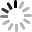#### Numpy库之符号函数sign()的介绍及用法

np.sign()是一个符号函数，用于把函数的符号析离出来，定义如下： python示例代码： #%% import numpy as np data = 1 print(data,'=>', np.sign(data)) data = 0 print(data,'=>', np.sign(data)) data = -1 print(data,'=>', np.sign(data)) data...

#### Python3.6.3 安装 numpy

NumPy系统是Python的一种开源的数值计算扩展。这种工具可用来存储和处理大型矩阵，比Python自身的嵌套列表（nested list structure)结构要高效的多（该结构也可以用来表示矩阵（matrix））。据说NumPy将Python相当于变成一种免费的更强大的MatLab系统。NumPy本身用C实现。 但是numpy对python3的支持不是很快，目pip支持最高python3...